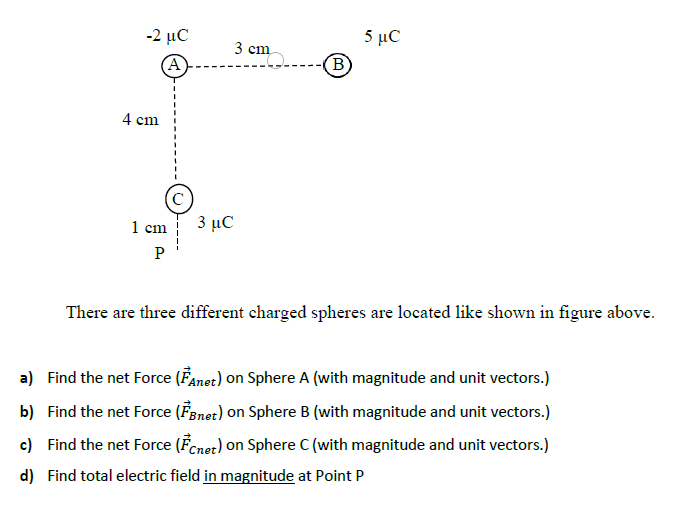# How Can I Solve Question Type: "With Magnitude and Unit Vectors"?

denfaro
Hi I am a beginner in this topic. I didn't understand this question type clearly.What does it mean" With Magnitude and Unit Vectors" exactly? May you help me for the solution step by step :). Thanks in advance.Mentor
Hi I am a beginner in this topic. I didn't understand this question type clearly.What does it mean" With Magnitude and Unit Vectors" exactly? May you help me for the solution step by step :). Thanks in advance.

View attachment 260846
Welcome to the PF.We cannot do your schoolwork/homework for you, but perhaps we can give you a few tips to help your understanding so that you can start working on the problem.

What have you done with vectors so far? You know that vectors have a magnitude and direction, right? And have you learned about coordinate systems like Caretesian coordinates, where there are x and y directions, etc.?

denfaro
Welcome to the PF.We cannot do your schoolwork/homework for you, but perhaps we can give you a few tips to help your understanding so that you can start working on the problem.

What have you done with vectors so far? You know that vectors have a magnitude and direction, right? And have you learned about coordinate systems like Caretesian coordinates, where there are x and y directions, etc.?
Yes I learned the vectors,coordinate system but I don't know how can I use them on this question.

Mentor
Yes I learned the vectors,coordinate system but I don't know how can I use them on this question.
So start by drawing an x-y set of axes on the diagram. I would probably make the (0,0) point at the A charge, with the x-axis to the right and the y-axis pointing up.

Then write the general vector force equation for the force on one charge due the electric field from another charge (based on the amount of the charges and the separation distance).

Please do those things, and show us what you get. Thank you.

••Yes I learned the vectors,coordinate system but I don't know how can I use them on this question.
Do you know charged spheres will apply force on each other?

denfaro
Do you know charged spheres will apply force on each other?
Yes I know

Yes I know
What’s the expression for that force? I mean is there any law which governs how two charged spheres will apply force on each other ?

denfaro
What’s the expression for that force? I mean is there any law which governs how two charged spheres will apply force on each other ?
I think you mean Coulmb's Law

I think you mean Coulmb's Law
Yes. Can you please write out the Coulombs Law?

denfaro
Yes. Can you please write out the Coulombs Law?
F=(k*(q1*q2))/r^2.

F=(k*(q1*q2))/r^2.
But force is a vector quantity, the expression which you have given doesn’t involve the direction of the force. Can you fix it? Can you do something so that we get a vector quantity in that expression of Coulombs Law?

denfaro
But force is a vector quantity, the expression which you have given doesn’t involve the direction of the force. Can you fix it? Can you do something so that we get a vector quantity in that expression of Coulombs Law?
Sorry I don't. How can I do that?

Sorry I don't. How can I do that?
$$\vec{F} = k \frac{q_1 ~q_2 }{r^2}~ \hat{r}$$ the force acts on the line joining the two charges.

••berkeman and denfaro
denfaro
$$\vec{F} = k \frac{q_1 ~q_2 }{r^2}~ \hat{r}$$ the force acts on the line joining the two charges.
Thanks I have an idea now. :)

•And it's important to remember that the force between like charges is repulsive, and the force between opposite charges is attractive. That's where the direction for the force vector comes from.